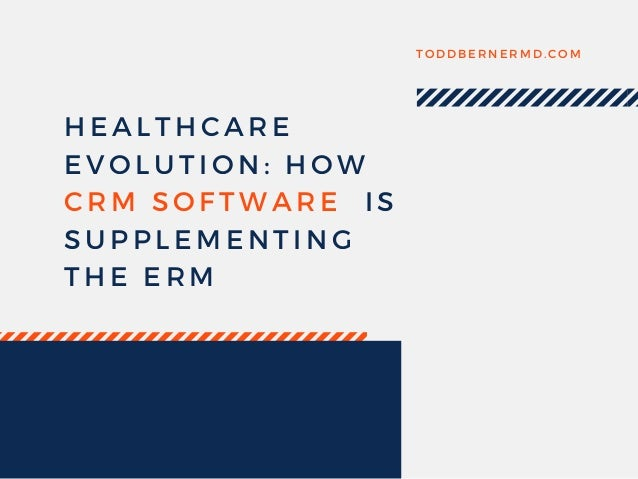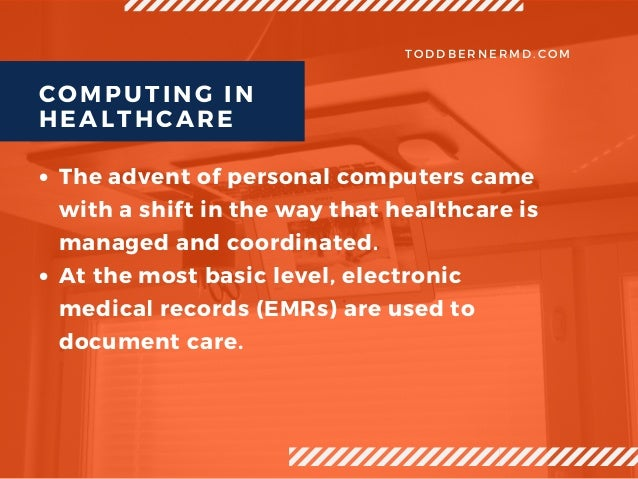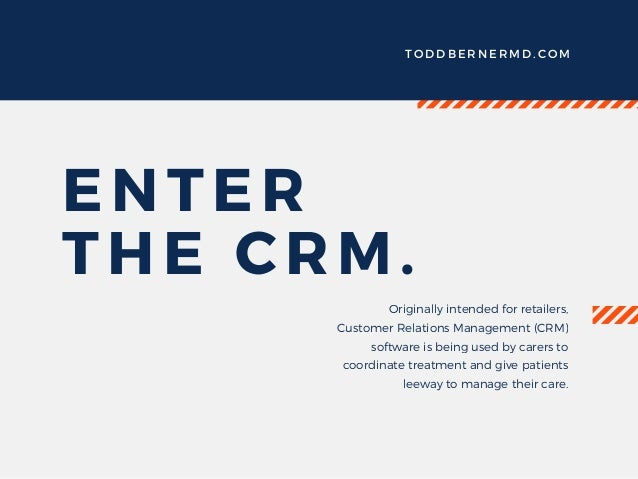Successfully reported this slideshow.Upcoming SlideShare
×

of0

Share

# Healthcare Evolution: How CRM Software is Supplementing the ERM

Todd Berner takes a look at customer relations management software, originally built for retailers and now useful in the healthcare industry. Read about the ways that this software can help carers engage with their patients.

See all

### Related Audiobooks

#### Free with a 30 day trial from Scribd

See all
• Be the first to like this

### Healthcare Evolution: How CRM Software is Supplementing the ERM

1. 1. H E A L T H C A R E E V O L U T I O N : H O W C R M S O F T W A R E   I S S U P P L E M E N T I N G T H E E R M T O D D B E R N E R M D . C O M
2. 2. T O D D B E R N E R M D . C O M The advent of personal computers came with a shift in the way that healthcare is managed and coordinated. At the most basic level, electronic medical records (EMRs) are used to document care. COMPUTING IN HEALTHCARE
3. 3. T O D D B E R N E R M D . C O M ENTER THE CRM. Originally intended for retailers, Customer Relations Management (CRM) software is being used by carers to coordinate treatment and give patients leeway to manage their care.
4. 4. T H E E R M I S N ' T G O I N G A W A Y , B U T C R M S O F T W A R E A L L O W S C A R E R S T O R E D U C E C O S T S A N D P R O V I D E B E T T E R C A R E . T O D D B E R N E R M D . C O M
5. 5. T O D D B E R N E R M D . C O M Improve patient communications Generate paperwork Provide analytics for marketing and research Automate appointment and referral processes WHAT CAN CRMS DO?
6. 6. C R M S O L U T I O N S O F F E R P A T I E N T S T H E C H A N C E T O S E E A C O M P L E T E R E P O R T O F T H E I R H E A L T H , B A S E D O N I N F O R M A T I O N F R O M A V A R I E T Y O F C A R E R S . T O D D B E R N E R M D . C O M H O W W I L L C R M S Y S T E M S I M P R O V E ? A N A L Y T I C S C A N I M P R O V E P O P U L A T I O N H E A L T H A N D R E S E A R C H I N I T I A T I V E S . H E A L T H   T R E N D S W I L L B E C O M E I N C R E A S I N G L Y E A S Y T O D E T E C T .
7. 7. C U R R E N T L Y , C R M S O F T W A R E I S A L R E A D Y A L L O W I N G F O R E A S I E R C O N T A C T W I T H M E D I C A L P R O F E S S I O N A L S A N D H E L P S P R O A C T I V E T R E A T M E N T . T O D D B E R N E R M D . C O M I T H A S A L R E A D Y D O N E A L O T O F G O O D , A T R E N D T H A T I S S U R E T O I M P R O V E I N T H E F U T U R E .
8. 8. T H A N K S F O R R E A D I N G ! R E A D T H E F U L L B L O G A T T O D D B E R N E R M D . C O M

Total views

539

On Slideshare

0

From embeds

0

Number of embeds

131

3

Shares

0